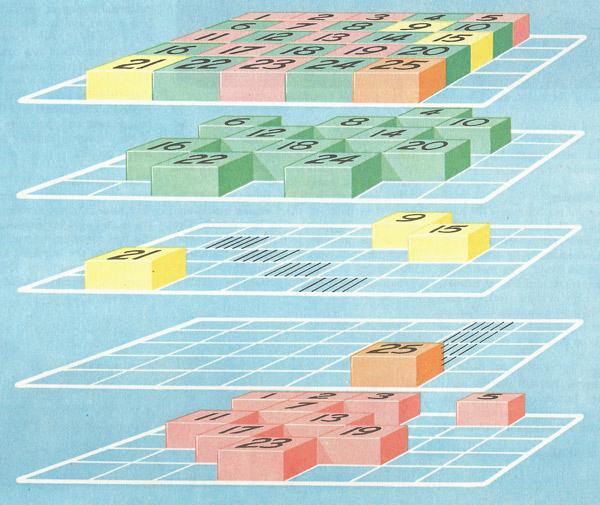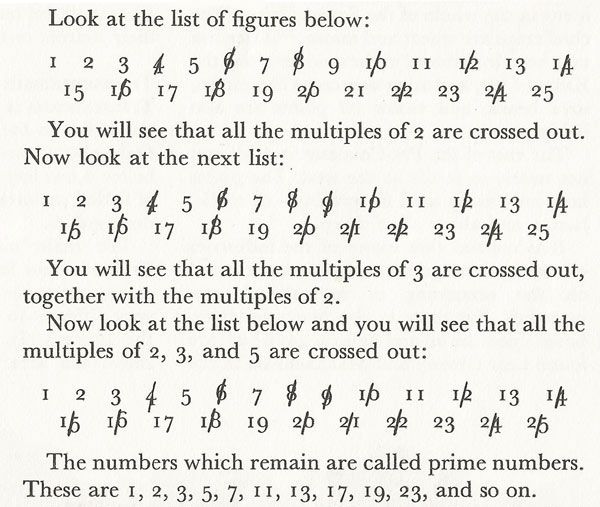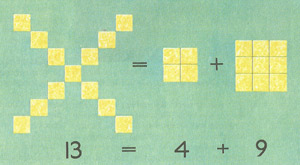A# prime numberFig 1. The sieve of Eratosthenes.Fig 2. An explanation of the sieve of Eratosthenes.Fig 3. Prime numbers that have a starred pattern, similar to the one frequently used to denote 5, can always be expressed as the sum of two square numbers.

A prime number is an integer greater than 1 that is divisible only by 1 and itself. The prime numbers are 2, 3, 5, 7, 11, 13, 17, 19, 23, 29, 31, 37, 41, 43, 47, 53, 59, 61, 67, 71, 73, 79, 83, 89, 97, ... Another way to think of them is as numbers that are not rectangular numbers (i.e. numbers that can be arranged in a rectangular form.

## Sieve of Eratosthenes

Eratosthenes, a Greek mathematician and a friend of Archimedes, devised a method for obtaining prime numbers in their numerical order. This method is known as the sieve of Eratosthenes. Fig 1 shows the principles on which it works.

Look at the drawing of the sieve. Imagine that all the numbers from 1 to 25 are being dropped through the sieve. All these numbers drop through the top rack, but on on the second those which are multiples of 2 are caught. These are colored green.

On the third rack all the numbers which are multiples of 3 are caught, except of course, those which have already been caught as multiples of two. These are colored yellow. In the fourth rack 25 is caught - this is the only multiple of 5 which is neither a multiple of 3 nor a multiple of 2. It is colored orange.

The final numbers that get through to the final rack are prime numbers (see Figure 2).

You will see that there are black squares on the sieve. In the third rack these show where the multiples of 3 would have been caught if they had not been caught by the rack above. The black squares in the fourth rack show where the multiples of 5 would have been caught had they not been caught by the two racks above.

You may wonder why there is no rack taking out multiples of 4. This is because 4 is a multiple of 2, and therefore all multiples of 4 are also multiples of 2.

## Formulae for primes

This 'sieve' method of producing prime numbers is laborious, and mathematicians have long been searching for a simple method of producing them, without success.

The expression n2 – n + 41 might appear to be a possible formula for prime numbers.

When n = 1 the expression = 41 which is prime
When n = 2 the expression = 43 which is prime
When n = 3 the expression = 47 which is prime
When n = 4 the expression = 53 which is prime
and so on. But it breaks down when n = 41. Can you see why?

Fermat, a seventeenth-century mathematician, thought that he had found a formula for prime numbers: 22^n + 1.

When n = 1 the expression = 5(22 + 1)
When n = 2 the expression = 17(24 + 1)
When n = 3 the expression = 257(28 + 1)
When n = 4 the expression = 65,537(216 + 1)
When n = 5 the expression = 4,294,967,297(232 + 1)

However, Euler, showed that the last number is not prime but is, in fact, 641 x 6,700,417.

## Largest prime known

It takes a long time to decide whether any number is prime, and to date, the largest one known is 282,589,933 – 1 which, when worked out has 24,862,048 digits. The testing was done on a computer.

## Number of primes

One may wonder whether the primes go on for ever or whether there is in fact one number which is the largest prime number. Euclid, by a very neat argument, proved that there are an unlimited number of primes.

Suppose, he said, that the largest prime number you have found is p. Multiply all the previous prime numbers including p together and add 1. If the result is a prime number, it is naturally, a larger prime than p. If not, it must have divisors none of which can be one of the original primes since they all leave a remainder 1 on division. The divisors of the new number, then, must be prime numbers greater than p. So in either case there is a prime number greater than p, and this process can be continued forever.

## Distribution of primes

Prime numbers have fascinated mathematicians for centuries, in large part because of how they are distributed: at first sight, they seem to occur randomly, and yet, on closer inspection, they reveal a subtle order or pattern, that seems to hold deep truths about the nature of mathematics and of the world in which we live. The German-born American mathematician Don Zagier (1951–), in his inaugural lecture at Bonn University, put it this way:

"There are two facts about the distribution of prime numbers which I hope to convince you... The first is that despite their simple definition and role as the building blocks of the natural numbers, the prime numbers ... grow like weeds among the natural numbers, seeming to obey no other law than that of chance, and nobody can predict where the next one will sprout. The second fact is even more astonishing, for it states just the opposite: that the prime numbers exhibit stunning regularity, that there are laws governing their behavior, and that they obey these laws with almost military precision."

## Properties of primes

Prime numbers have many interesting properties. For instance, those which have a starred pattern, similar to the one frequently used to denote 5, can always be expressed as the sum of two square numbers.

The fundamental theorem of arithmetic declares that the primes are the building blocks of the positive integers: every positive integer is a product of prime numbers in one and only one way, except for the order of the factors. This is the key to their importance: the prime factors of an integer determines its properties. The ancient Greeks proved (c. 300 BC) that there are infinitely many primes and that they are irregularly spaced; in fact, there can be arbitrarily large gaps between successive primes. On the other hand, in the 19th century it was shown that the number of primes less than or equal to n approaches n / log n, as n gets very large (a result known as the prime number theorem), so that a rough estimate for the nth prime is n log n. In his Disquisitiones Arithmeticae (1801), Carl Gauss wrote:

"The problem of distinguishing prime numbers from composite numbers and of resolving the latter into their prime factors is known to be one of the most important and useful in arithmetic. It has engaged the industry and wisdom of ancient and modern geometers to such an extent that it would be superfluous to discuss the problem at length... Further, the dignity of the science itself seems to require that every possible means be explored for the solution of a problem so elegant and so celebrated."

The earliest known primality test, as discussed earlier, is the sieve of Eratosthenes, which dates from around 240 BC. However, the use of high-speed computers and of fast algorithms are needed to identify large primes. New record-breaking primes tend to be of the variety known as Mersenne primes, since these are the easiest to find. Much remains unknown about the primes. As Martin Gardner said:

"No branch of number theory is more saturated with mystery ... Some problems concerning primes are so simple that a child can understand them and yet so deep and far from solved that many mathematicians now suspect they have no solution. Perhaps they are 'undecidable.' Perhaps number theory, like quantum mechanics, has its own uncertainty principle that makes it necessary, in certain areas, to abandon exactness for probabilistic formulations."

One of the greatest unsolved problems in mathematics, the Riemann hypothesis, the distribution of prime numbers. There are also some very simple properties of prime numbers which have not yet been proved. A famous example, known as Goldbach's conjecture, is that any number can be expressed as the sum of two primes:

12 = 5 + 7
20 = 17 + 3
34 = 17 + 17 and so on

Nobody has, so far, been able to find out why this should be so, and yet no one has found an even number which cannot be split into two primes.

It has also been proposed that there are an infinite number of so-called prime pairs which are prime numbers differing by two. (11, 13), (29, 31) are cases, but again, no one has yet to establish whether this is so.

## Types of prime number

### circular prime

A circular prime is a prime number that remains prime on any cyclic rotation of its digits. An example (in the decimal system) is 1193 because 1931, 9311, and 3119 are also prime. Any one-digit prime is circular by default. In base ten, any circular prime with two or more digits can only contain the digits 1, 3, 7 and 9; otherwise when 0, 2, 4, 5, 6, or 8 is rotated into the units place, the result will be divisible by 2 or 5. The only circular primes known, if we list by just the smallest representative from each cycle, are:

2, 3, 5, 7, 11, 13, 17, 37, 79, 113, 197, 199, 337, 1193, 3779, 11939, 19937, 193939, 199933, R19, R23, R317, R1031 and possibly R49081.

These last five are the known repunit primes and probable primes. It is conjectured that there are infinitely many repunit primes, so there should be infinitely many circular primes. But it is highly likely that all circular primes not on the list above are repunits.

### coprime

Two or more numbers are coprime if they have no factors in common other than 1.

### Cunningham chain

A Cunningham chain is a sequence of prime numbers in which each member is twice the previous one plus one. For example, {2, 5, 11, 23, 47} is the first Cunningham chain of length 5 and {89, 179, 359, 719, 1439, 2879} is the first of length 6. More generally, a Cunningham chain of length k of the first kind is a sequence of k prime numbers, each of which is twice the preceding one plus one. A Cunningham chain of length k of the second kind is a sequence of k primes, each of which is twice the preceding one minus one. For example, {2, 3, 5} is a Cunningham chain of length 3 of the second kind and {1531, 3061, 6121, 12241, 24481} is a Cunningham chain of length 5 of the second kind. Primes of both these forms are called complete chains if they can't be extended by adding either the next larger or the next smaller terms. See also Sophie Germain prime.

### emirp

An emirp is prime number that becomes a different prime number when its digits are reversed ("emirp" is "prime" spelled backwards). The first twenty emirps are 13, 17, 31, 37, 71, 73, 79, 97, 107, 113, 149, 157, 167, 179, 199, 311, 337, 347, 359, and 389. Compare with a palindromic prime (see palindromic number), which gives the same prime when reversed.

### Mersenne prime

A Mersenne prime is a prime number of the form 2p – 1, where p is prime.

### minimal prime

Every prime number, when written in base ten, has one of the following primes as a substring:

2, 3, 5, 7, 11, 19, 41, 61, 89, 409, 449, 499, 881, 991, 6469, 6949, 9001, 9049, 9649, 9949, 60649, 666649, 946669, 60000049, 66000049, 66600049

These are the minimal primes. A string a is a substring of another string b, if a can be obtained from b by deleting zero or more of the characters in b. For example, 392 is a substring of 639802.

### permutable prime

A permutable prime, also known as an absolute prime, is a prime number with at least two distinct digits which remains prime on every rearrangement (permutation) of the digits. For example, 337 is a permutable prime because each of 337, 373, and 733 are prime. Most likely, in base ten, the only permutable primes are 13, 17, 37, 79, 113, 199, 337, and their permutations. Obviously permutable primes cannot have any of the digits 2, 4, 6, 8 or 5. They also cannot have all four of the digits 1, 3, 7, and 9 simultaneously.

### pseudoprime

A pseudoprime is a number that passes the test of Fermat's little theorem (FLT) for prime numbers but actually isn't a prime. FLT says that if p is prime and a is coprime to p, then a p–1 – 1 is divisible by p. If a number x is not prime, a is coprime to x, and x divides a x–1 – 1, then x is called a pseudoprime to base a.

A number x that is a pseudoprime for all values of a that are coprime to x is called a Carmichael number. The smallest pseudoprime for in base 2 is 341. It isn't prime because 341 = 11 × 31; however, it satisfies FLT: 2340 – 1 is divisible by 341.

### snowball prime

A snowball prime also known as a right-truncatable prime, is a prime number whose digits can be chopped off, one by one, from the right-hand side, yet still leave a prime number. This means that even if you stop writing before you finish the number, you will still have written a prime. The largest snowball prime is 73939133 (7, 73, 739, ..., 73939133 are all prime).

### Sophie Germain prime

A Sophie Germain prime is any prime number p such that 2p + 1 is also prime; the smallest examples are 2, 3, 5, 11, 23, 29, 41, 53, 83, 89, 113, and 131. Around 1825 Sophie Germain proved that the first case of Fermat's last theorem is true for such primes. Soon after, Adrien-Marie Legendre began to generalize this by showing the first case of Fermat's last theorem also holds for odd primes p such that kp + 1 is prime, k = 4, 8, 10, 14, and 16. In 1991 Fee and Granville extended this to k < 100, where k not a multiple of three. Many similar results were also shown, but now that Fermat's last theorem has been proven correct, they are of less interest.

### strobogrammatic prime

A strobogrammatic prime is a prime number that remains unchanged when rotated through 180°. An example is 619, which looks the same when read upside-down. To be strobogrammatic, a prime cannot contain digits other than 0, 1, and 8, which have a horizontal line of symmetry (ignoring font variations), and 6 and 9, which are vertical reflections of each other. An invertible prime is one that yields a different prime when the digits are inverted. Of course, these definitions are not taken seriously by mathematicians!

### truncatable prime

A truncatable prime is a prime number n that remains a prime when digits are deleted from it one at a time. For example 410256793 is a truncatable prime because each number created by the removal of the digit underlined produces a new prime: 410256793, 41256793, 4125673, 415673, 45673, 4567, 467, 67, 7. It is conjectured that there are infinitely many of these primes. If the digits from a prime can be deleted only from the right to leave a prime, then n is called a right truncatable prime. If they can be deleted only from the left to leave a prime, then n is a left truncatable prime. The list of primes from which any digit can be deleted at each step to leave a prime is very short indeed, because it demands that each digit be a prime and also that no digit occurs twice. Only these numbers satisfy this requirement: 2, 3, 5, 7, 23, 37, 53 and 73.

### twin primes

Twin primes are pairs of prime numbers that differ by two, the first of which are 3 and 5, 5 and 7, 11 and 13.

## Other quantities related to primes

### primitive root

A primitive root for a prime number p is one whose powers generate all the non-zero integers modulo p. For example, 3 is a primitive root modulo 7 since 3 = 31, 2 = 32 mod 7, 6 = 33 mod 7, 4 = 34 mod 7, 5 = 35 mod 7, 1 = 36 mod 7.

### primorial

A primorial, also known as a prime factorial, is the product of all prime numbers that are less than or equal to a given prime p; it is denoted p#. For example 3# = 2 × 3 = 6, 5# = 2 × 3 × 5 = 30, and 13# = 2 × 3 × 5 × 7 × 11 × 13 = 30030.

## Primes and the hunt for alien intelligence

In 1941, James Jeans pointed out that the attention of intelligent Martians "if any such there be" could be attracted by using powerful searchlights to flash, in sequence, the first prime numbers: 2, 3, 5, 7, 11, 13, 17, 19, 23, ... Likewise, it has been suggested that the occurrence of sequences of prime numbers in an extraterrestrial radio signal would immediately imply an artificial and intelligent origin. The reception of such a signal occurs in the novel (and film) Contact. Prime numbers were also used in the construction of Drake's cryptogram and the Arecibo message.